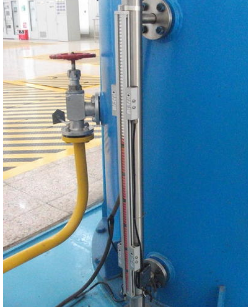# 一、背景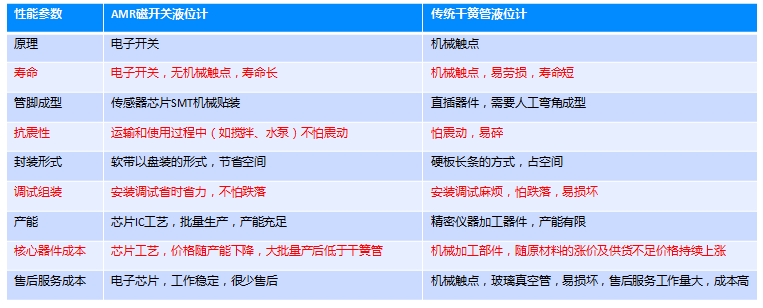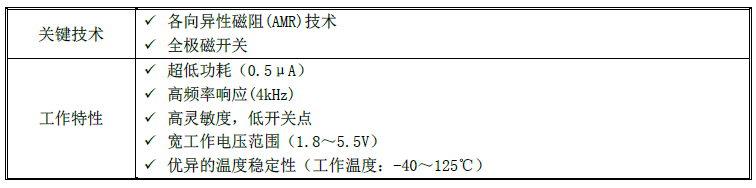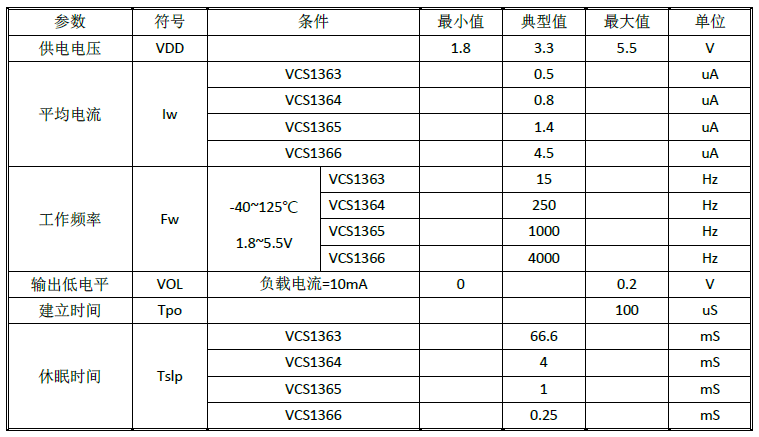­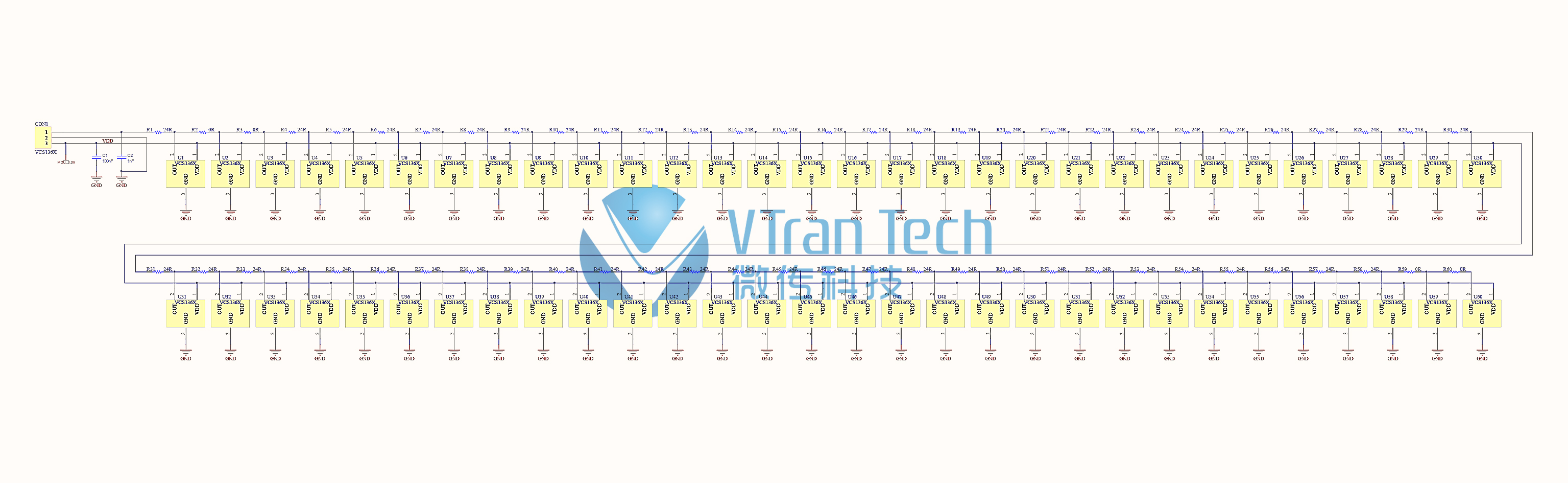4 液位计30cm原理图参考设计电路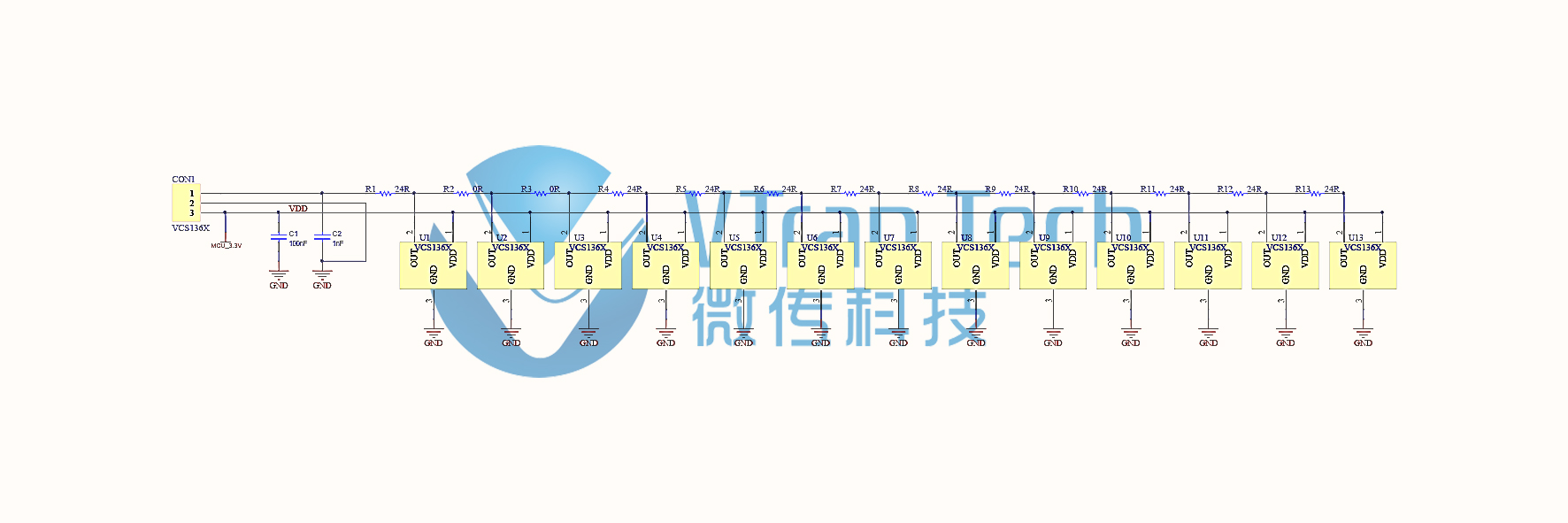1）VCS136X电阻网络，如上图所示。13个VCS136X共电源VDD，共地GND。

2）Vout和 GND之间的阻值为 Rs，由 VCS136X 和电阻组成的电路确定。

3）在VCS136X感应到磁场时，VCS136X中输出引脚（VOUT）和地（GND）导通。

4）随磁场位置移动，U1~U13依次导通和断开，RS阻值发生变化如下：

U1导通时其它断开，RS=R1。

U2导通时其它断开，RS=R1+R2。

U3导通时其它断开，RS=R1+R2+R3。

U4导通时其它断开，RS=R1+R2+R3+R4。

U5导通时其它断开，RS=R1+R2+R3+R4+R5。

U6导通时其它断开，RS=R1+R2+R3+R4+R5+R6。

U7导通时其它断开，RS=R1+R2+R3+R4+R5+R6+R7。

U8导通时其它断开，RS=R1+R2+R3+R4+R5+R6+R7+R8。

U9导通时其它断开，RS=R1+R2+R3+R4+R5+R6+R7+R8+R9。

U10导通时其它断开，RS=R1+R2+R3+R4+R5+R6+R7+R8+R9+R10。

U11导通时其它断开，RS= R1+R2+R3+R4+R5+R6+R7+R8+R9+R10+R11。

U12导通时其它断开，RS=R1+R2+R3+R4+R5+R6+R7+R8+R9+R10+R11+R12。

U13导通时其它断开，RS=R1+R2+R3+R4+R5+R6+R7+R8+R9+R10+R11+R12+R13。

（其中R2和R3为0欧姆，前面三颗位置为起始调零位，防止跳变）